×
INTELLIGENT WORK FORUMS
FOR ENGINEERING PROFESSIONALS

Are you an
Engineering professional?
Join Eng-Tips Forums!
• Talk With Other Members
• Be Notified Of Responses
• Keyword Search
Favorite Forums
• Automated Signatures
• Best Of All, It's Free!

*Eng-Tips's functionality depends on members receiving e-mail. By joining you are opting in to receive e-mail.

#### Posting Guidelines

Promoting, selling, recruiting, coursework and thesis posting is forbidden.

# Python Solve Functions - Help For A Noobie2

## Python Solve Functions - Help For A Noobie

(OP)
sparked by the python coding discussion by Celt83, I am diving in and trying to solve instantaneous center of rotation problems with python.

The example problem I am trying to solve is outlined here:
https://engineering.purdue.edu/~jliu/courses/CE591...

The way I see this problem, there are (3) unknowns:
1. X location of the instantaneous center of rotation,
1. Y location of the instantaneous
(sorry don't know how to fix the numbering above, fiddled for too damn long)

I also have (3) equations that need to equal 0
• Sum of Forces in the X = 0
• Sum of Forces in the Y = 0
• Sum of Moments = 0

Even in my "native coding language" matlab, this would be somewhat challenging to code, so I am sure it will be fun using this as a learning experience in python.

Anyways, python experts, do you have any suggestions on how to solve this? Should I explore symbolic notation within in python? Should I use a solve block that iterates through the (3) unknowns?

Any help or suggestions/starting points would be appreciated.

My base code that does the guess and check version of this is shown below: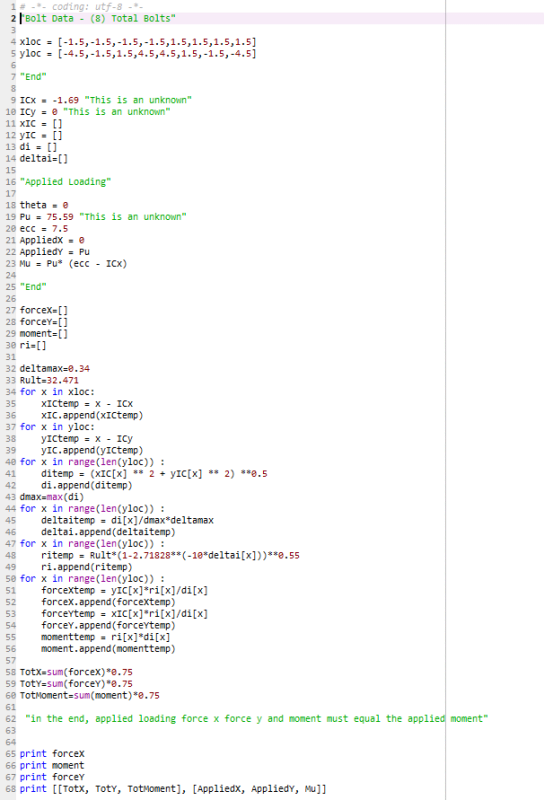The final print shows the comparison of applied loading verse resist force.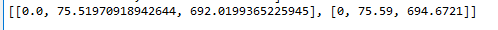S&T

### RE: Python Solve Functions - Help For A Noobie

The Scipy library has several solver functions that will do what you want.

https://newtonexcelbach.com/2018/10/21/iterative-s...

The spreadsheet calls the Python functions from Excel using xlwings, but if you prefer to work directly with Python the Python code is included in the download.

For solvers with more than one unknown see the Solvers2 sheet, which use the Scipy.Optimize minimize function. You need to write a function that returns the difference between calculated and target values for given inputs, with output as a Numpy array, and the minimize function will adjust the inputs to minimize the differences.

Doug Jenkins
Interactive Design Services
http://newtonexcelbach.wordpress.com/

### RE: Python Solve Functions - Help For A Noobie

(OP)
IDS, thank you.

Feel a little overwhelmed currently, lots of learning to ensue...

S&T

### RE: Python Solve Functions - Help For A Noobie

Let us know how you go.

To use Scipy you will also need Numpy, but they are both pretty well indispensable for engineering work with Python.

As an example of what you can do with Numpy, using the xloc list from your code:

#### CODE --> Python

import numpy as np
…
# Convert xloc to a Numpy array
xloc = np.array(xloc)
ICX = -1.69
# Subtract ICX from all xloc values in one operation
xIC = xloc - ICX 

Doug Jenkins
Interactive Design Services
http://newtonexcelbach.wordpress.com/

### RE: Python Solve Functions - Help For A Noobie

hmm learned something new hadn't ever looked into Scipy for this, here's my code below which seems to check out:
It is pretty sensitive to where the initial IC coordinates are assumed to be

As IDS notes you can condense a lot of this down to single line steps using numpy arrays, I kept the bulk of it as native python lists and broken up into the calculation steps.

#### CODE --> python

from __future__ import division
import math as m
import scipy.optimize as sci

def ic_check(IC,xloc,yloc, ecc, theta):
num_bolts = len(xloc)

deltamax = 0.34
Rult = 32.471

ICx = IC
ICy = IC
xIC = []
yIC = []
di = []
deltai = []
ri = []

fx = []
fy = []
moment = []

for x in xloc:
xICtemp = x - ICx
xIC.append(xICtemp)

for y in yloc:
yICtemp = y - ICy
yIC.append(yICtemp)

i=0
for i in range(num_bolts):
ditemp = m.sqrt((xIC[i]*xIC[i])+(yIC[i]*yIC[i]))
di.append(ditemp)

dmax = max(di)

i=0
for i in range(num_bolts):
deltaitemp = (di[i]/dmax)*deltamax
deltai.append(deltaitemp)

i=0
for i in range(num_bolts):
ritemp = Rult*m.pow(1-m.pow(m.e,-10.0*deltai[i]),0.55)
ri.append(ritemp)

i=0
for i in range(num_bolts):
fxtemp = (yIC[i]*ri[i])/di[i]
fx.append(fxtemp)

i=0
for i in range(num_bolts):
fytemp = (xIC[i]*ri[i])/di[i]
fy.append(fytemp)

i=0
for i in range(num_bolts):
momenttemp = ri[i]*di[i]
moment.append(momenttemp)

totX = sum(fx)*0.75
totY = sum(fy)*0.75
totM = sum(moment)*0.75

Pu_force = m.sqrt((totX*totX) + (totY*totY))

res1 = [totX, totY, totM]

res2 = [Pu_force, Pu_moment]

return res1, res2

def ic_optimize(IC,xloc,yloc,ecc,theta):

res1, res2 = ic_check(IC,xloc,yloc,ecc,theta)

res = res2 - res2

return abs(res)

xloc = [-1.5,-1.5,-1.5,-1.5,1.5,1.5,1.5,1.5]
yloc = [-4.5,-1.5,1.5,4.5,4.5,1.5,-1.5,-4.5]

ICx = -1.69
ICy = 0.0
IC = [ICx,ICy]
theta = 0.0
ecc = 7.5
res1, res2 = ic_check(IC,xloc,yloc,ecc,theta)

test2 = ic_optimize(IC,xloc,yloc,ecc,theta)

test = sci.minimize(ic_optimize,IC,(xloc,yloc,ecc,theta), tol=1e-6)

IC_new = [test.x,test.x]

res3, res4 = ic_check(IC_new,xloc,yloc,ecc,theta) 

Open Source Structural Applications: https://github.com/buddyd16/Structural-Engineering

### RE: Python Solve Functions - Help For A Noobie

I improved the results slightly by also back checking sum Fx and sum Fy in my optimization function:
It is still very sensitive to the initial IC coordinate guess.

There is a great article on AISC for this by G. Donald Brandt from 2nd quarter 1982: https://www.aisc.org/Rapid-Determination-of-Ultima...

#### CODE --> python

def ic_optimize(IC,xloc,yloc,ecc,theta):

res1, res2, check = ic_check(IC,xloc,yloc,ecc,theta)

res = res2 - res2

fx = px + res1
fy = py - res1

res_magnitude = m.sqrt(res*res+fx*fx+fy*fy)

return res_magnitude 

Open Source Structural Applications: https://github.com/buddyd16/Structural-Engineering

### RE: Python Solve Functions - Help For A Noobie

Brandt's Method is much more reliable:

#### CODE --> python

from __future__ import division
import math as m
import scipy.optimize as sci

def ic_check(IC,xloc,yloc, ecc, theta):
num_bolts = len(xloc)

deltamax = 0.34
Rult = 32.471

ICx = IC
ICy = IC
xIC = []
yIC = []
di = []
deltai = []
ri = []

fx = []
fy = []
moment = []

for x in xloc:
xICtemp = x - ICx
xIC.append(xICtemp)

for y in yloc:
yICtemp = y - ICy
yIC.append(yICtemp)

i=0
for i in range(num_bolts):
ditemp = m.sqrt((xIC[i]*xIC[i])+(yIC[i]*yIC[i]))
di.append(ditemp)

dmax = max(di)

i=0
for i in range(num_bolts):
deltaitemp = (di[i]/dmax)*deltamax
deltai.append(deltaitemp)

i=0
for i in range(num_bolts):
ritemp = Rult*m.pow(1-m.pow(m.e,-10.0*deltai[i]),0.55)
ri.append(ritemp)

i=0
for i in range(num_bolts):
fxtemp = (yIC[i]*ri[i])/di[i]
fx.append(fxtemp)

i=0
for i in range(num_bolts):
fytemp = (xIC[i]*ri[i])/di[i]
fy.append(fytemp)

i=0
for i in range(num_bolts):
momenttemp = ri[i]*di[i]
moment.append(momenttemp)

totX = sum(fx)*0.75
totY = sum(fy)*0.75
totM = sum(moment)*0.75

Pu_force = m.sqrt((totX*totX) + (totY*totY))

res1 = [totX, totY, totM]

res2 = [Pu_force, Pu_moment]

check_output = [xIC,yIC,di,deltai,ri,fx,fy]

return res1, res2, check_output

def ic_optimize(IC,xloc,yloc,ecc,theta):

res1, res2, check = ic_check(IC,xloc,yloc,ecc,theta)

res = res2 - res2

fx = px - res1
fy = py - res1

res_magnitude = m.sqrt(res*res+fx*fx+fy*fy)

return res_magnitude

def ic_brandt(IC, xloc, yloc, Mp):
num_bolts = len(xloc)

deltamax = 0.34

ICx = IC
ICy = IC
xIC = []
yIC = []
di = []
deltai = []
ri = []

fx = []
fy = []
moment = []

for x in xloc:
xICtemp = x - ICx
xIC.append(xICtemp)

for y in yloc:
yICtemp = y - ICy
yIC.append(yICtemp)

i=0
for i in range(num_bolts):
ditemp = m.sqrt((xIC[i]*xIC[i])+(yIC[i]*yIC[i]))
di.append(ditemp)

dmax = max(di)

i=0
for i in range(num_bolts):
deltaitemp = (di[i]/dmax)*deltamax
deltai.append(deltaitemp)

i=0
for i in range(num_bolts):
ritemp = m.pow(1-m.pow(m.e,-10.0*deltai[i]),0.55)
ri.append(ritemp)

i=0
for i in range(num_bolts):
fxtemp = -1*(yIC[i]*ri[i])/di[i]
fx.append(fxtemp)

i=0
for i in range(num_bolts):
fytemp = (xIC[i]*ri[i])/di[i]
fy.append(fytemp)

i=0
for i in range(num_bolts):
momenttemp = ri[i]*di[i]
moment.append(momenttemp)

Mi = sum(moment)
Rult = -1*Mp/Mi

Rx = sum(fx) * Rult
Ry = sum(fy) * Rult

table = [xIC, yIC, di, deltai, ri, moment, fx, fy]

return Rx, Ry, Mi, table

def brandt(xloc, yloc, P_xloc, P_yloc, P_angle):
# Bolt Group Instantaneous Center using method by G. Donald Brandt
# Rapid Determiniation of Ultimate Strength Of Eccentrically Loaded Bolt Groups
# AISC Journal 1982 2nd Quarter

detailed_output = []

num_bolts = len(xloc)

n = num_bolts

detailed_output.append(num_bolts)

#Bolt Group Centroid
if len(xloc)<3:
anchor_x_bar = (xloc+xloc)/2.00
anchor_y_bar = (yloc+yloc)/2.00

else:
j=0
x_tot=0
y_tot=0

for i in xloc:
x_tot = x_tot+xloc[j]
y_tot = y_tot+yloc[j]
j+=1

anchor_x_bar = x_tot/len(xloc)
anchor_y_bar = y_tot/len(yloc)

cg_anchors = [anchor_x_bar, anchor_y_bar]
detailed_output.append(cg_anchors)

# J - Polar Moment of Inertial of Bolt Group
# sum(x^2+y^2)
sum_x_square = 0
sum_y_square = 0

i=0
for i in range(num_bolts):
sum_x_square = sum_x_square + (xloc[i]-anchor_x_bar)**2
sum_y_square = sum_y_square + (yloc[i]-anchor_y_bar)**2

J = sum_x_square + sum_y_square
detailed_output.append(['J',J])

detailed_output.append([Px,Py])

Mo = (-1*Px*(P_yloc-anchor_y_bar))+(Py*(P_xloc-anchor_x_bar))

detailed_output.append(Mo)

ax = (-1*Py*J) / (n * Mo)
ay = (Px*J) / (n*Mo)

detailed_output.append([ax,ay])

Mp = (-1*Px*(P_yloc-anchor_y_bar-ay))+(Py*(P_xloc-anchor_x_bar-ax))

detailed_output.append(Mp)

IC_initial = [anchor_x_bar+ax,anchor_y_bar+ay]

Rx, Ry, Mi, table = ic_brandt(IC_initial,xloc,yloc, Mp)

detailed_output.append([Rx, Ry, Mi, table,"First IC pass"])

fxx = Px + Rx
fyy = Py + Ry
F = m.sqrt(fxx*fxx+fyy*fyy)

detailed_output.append([fxx,fyy,F,"F"])

ax_new = (-1*fyy*J)/(n*Mo)
ay_new = (fxx*J) / (n*Mo)

detailed_output.append(["ax",ax_new,"ay",ay_new])

IC_new = IC_initial

count = 0
iterations = 0
while count<1000:

IC_new = [IC_new+ax_new,IC_new+ay_new]
Mp_new = (-1*Px*(P_yloc-IC_new))+(Py*(P_xloc-IC_new))

Rx, Ry, Mi, table = ic_brandt(IC_new,xloc,yloc, Mp_new)

fxx = Px + Rx
fyy = Py + Ry
F = m.sqrt(fxx*fxx+fyy*fyy)

ax_new = (-1*fyy*J)/(n*Mo)
ay_new = (fxx*J) / (n*Mo)

if F <= 0.00001:
iterations = count
count = 1000
solution = 'yes'
else:
count +=1
solution = 'no'

detailed_output.append([fxx,fyy,F])
detailed_output.append(IC_new)
detailed_output.append([solution,iterations,count])

detailed_output.append([Rx, Ry, Mi, table])

Cu = abs(Mi/Mp_new)

detailed_output.append([Mi,Mp_new,Cu])

return detailed_output, IC_new, Cu

# From Scratch Method Testing Zone
xloc = [-1.5,-1.5,-1.5,-1.5,1.5,1.5,1.5,1.5]
yloc = [-4.5,-1.5,1.5,4.5,4.5,1.5,-1.5,-4.5]

#Center of Anchor Group
if len(xloc)<3:
anchor_x_bar = (xloc+xloc)/2.00
anchor_y_bar = (yloc+yloc)/2.00

else:
j=0
x_tot=0
y_tot=0

for i in xloc:
x_tot = x_tot+xloc[j]
y_tot = y_tot+yloc[j]
j+=1

anchor_x_bar = x_tot/len(xloc)
anchor_y_bar = y_tot/len(yloc)

ICx = anchor_x_bar
ICy = anchor_y_bar
IC = [ICx,ICy]
theta = 0
ecc = 4
res1, res2, check1 = ic_check(IC,xloc,yloc,ecc,theta)

test2 = ic_optimize(IC,xloc,yloc,ecc,theta)

IC_new = [test.x,test.x]

res3, res4, check2 = ic_check(IC_new,xloc,yloc,ecc,theta)

C = res4/(0.75*32.471)

# Brandt's Method Testing Zone
# angle is positive counter-clockwise and measured from 0 pointing to the right
# anchor and P coordinates are global
x_b = [-1.5,-1.5,-1.5,-1.5,1.5,1.5,1.5,1.5]
y_b = [-4.5,-1.5,1.5,4.5,4.5,1.5,-1.5,-4.5]
P_xloc = 7
P_yloc = 0
P_angle = 90

brandt = brandt(x_b, y_b, P_xloc, P_yloc, P_angle) 

Open Source Structural Applications: https://github.com/buddyd16/Structural-Engineering

### RE: Python Solve Functions - Help For A Noobie

(OP)
thank you a ton Celt.

A few questions for you as I am trying to parse this code.

What does return do in python:

#### CODE --> Python

momenttemp = ri[i]*di[i]
moment.append(momenttemp)

totX = sum(fx)*0.75
totY = sum(fy)*0.75
totM = sum(moment)*0.75

Pu_force = m.sqrt((totX*totX) + (totY*totY))

res1 = [totX, totY, totM]

res2 = [Pu_force, Pu_moment]

check_output = [xIC,yIC,di,deltai,ri,fx,fy]

return res1, res2, check_output 

It looks like the program now knows the variables res1, res2, and check_output after the function is performed. Is this correct?

Could the (2) functions you have created be combined into one function? It looks like ic_optomize just builds on results from ic_check? The way I am interrupting this, res_magnitude (the final output from ic_optomize) could be programmed into ic_check.

Could the minimize function then be run ic_check in this case? Or does the function that is being minimized only allowed to have (1) output?

S&T

### RE: Python Solve Functions - Help For A Noobie

(OP)
i will also dig into the Brandt method when I get back to the office to login into AISC.

Looks like nice method for quick convergence.

S&T

### RE: Python Solve Functions - Help For A Noobie

The return line at the end of the function specifies what variable or value can be assigned as the function result to be used globally for further operations.

I have limited knowledge of how to properly use the scipy.optimize.minimize function but it seemed to want one result to check against, i also found that if the result was negative it would keep going negative rather than adjust to start returning back closer to 0.

Open Source Structural Applications: https://github.com/buddyd16/Structural-Engineering

### RE: Python Solve Functions - Help For A Noobie

You can probably implement Brandt's method in a spreadsheet and get reasonable results in 2 or 3 iterations without too much effort.

Open Source Structural Applications: https://github.com/buddyd16/Structural-Engineering

### RE: Python Solve Functions - Help For A Noobie

put together a GUI if your interested:
red dot is the IC
green dots are the bolts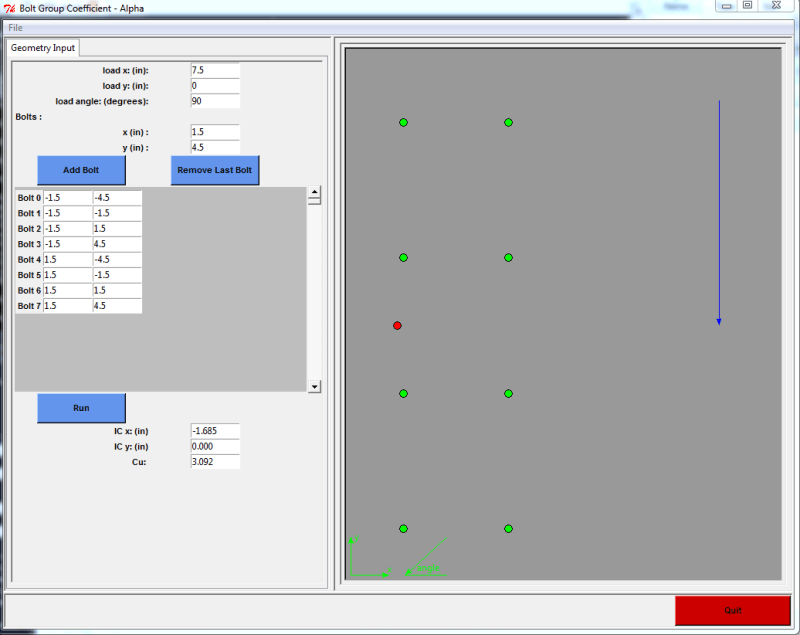Open Source Structural Applications: https://github.com/buddyd16/Structural-Engineering

### RE: Python Solve Functions - Help For A Noobie

(OP)
Cool stuff Celt! Digging into the GUI stuff this weekend once I get some free time.

S&T

### RE: Python Solve Functions - Help For A Noobie

I have now set this up to run using the Scipy.optimize root function:

#### CODE --> Python

def xlic_calc(IC, xloc, yloc, vals):
ecc =vals
deltamax = vals
Rult = vals
num_bolts = len(xloc)

ICx = IC
ICy = IC
Pu = IC
Pux = Pu * np.sin(theta)
Puy = Pu * np.cos(theta)
Mu = Pu * (ecc-ICx*np.cos(theta)-ICy*np.sin(theta))
xIC = []
yIC = []
di = []
deltai = []
ri = []

fx = []
fy = []
moment = []

for x in xloc:
xICtemp = x - ICx
xIC.append(xICtemp)

for y in yloc:
yICtemp = y - ICy
yIC.append(yICtemp)

i=0
for i in range(num_bolts):
ditemp = np.sqrt((xIC[i]*xIC[i])+(yIC[i]*yIC[i]))
di.append(ditemp)

dmax = max(di)

i=0
for i in range(num_bolts):
deltaitemp = (di[i]/dmax)*deltamax
deltai.append(deltaitemp)

ritemp = Rult*(1-np.e**(-10.0*deltai[i]))**0.55
ri.append(ritemp)

fxtemp = (yIC[i]*ri[i])/di[i]
fx.append(fxtemp)

fytemp = (xIC[i]*ri[i])/di[i]
fy.append(fytemp)

momenttemp = ri[i]*di[i]
moment.append(momenttemp)

totX = sum(fx)*0.75
totY = sum(fy)*0.75
totM = sum(moment)*0.75

return [totX, totY, totM, Pux, Puy, Mu] 

It's set up to be called from Excel using xlwings, but you can also call direct from Python:

>>> res = sopt.root(ic_check, x0, vals2)
>>> res.x
array([-1.68496865e+00, 4.01869965e-16, 7.53006559e+01])

Doug Jenkins
Interactive Design Services
http://newtonexcelbach.wordpress.com/

### RE: Python Solve Functions - Help For A Noobie

continued:
Inputs are:
x0, the initial guess: [-3, 0,100]
vals2: [[target vals],[[Xloc],[Yloc],[knowns]]]

[target vals] = [0,0,0]
[knowns] = [Eccentricity, Theta, deltamax,Rult]

I didn't have any problem with sensitivity to the initial guess values, and it works very fast with the default solver. Several of the other solvers work very slowly, or not at all.

I will post the Excel version in the next few days. Here's what it looks like: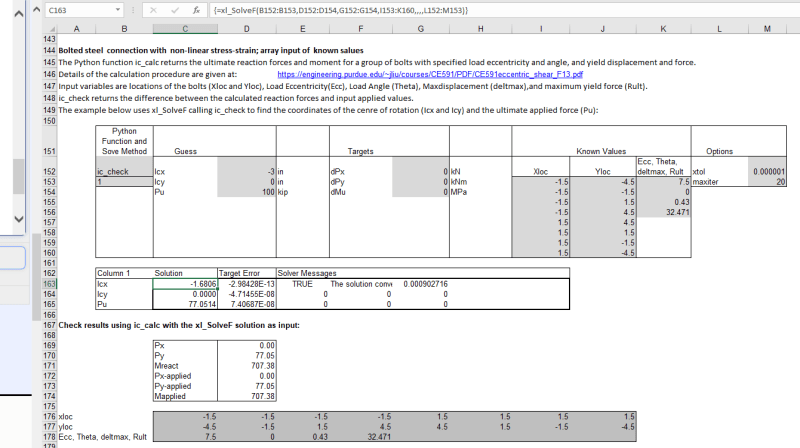Doug Jenkins
Interactive Design Services
http://newtonexcelbach.wordpress.com/

### RE: Python Solve Functions - Help For A Noobie

Part 3.

The ic_check function, which is the one you call from sopt.root:

#### CODE --> Python

def ic_check(IC, vals):
xloc = vals
yloc  = vals
vals2 =vals

res1 = xlic_calc(IC,xloc, yloc, vals2)

totX = res1
totY = res1
totM = res1

Pu =IC
Pux = res1
Puy = res1
Mu = res1

res3 = [totX-Pux, totY-Puy, totM-Mu]

return res3 

Doug Jenkins
Interactive Design Services
http://newtonexcelbach.wordpress.com/

### RE: Python Solve Functions - Help For A Noobie

@IDS:
I run into some problems with loads at flatter angles and small eccentricities, do you get the same issue. As an example try the load at an eccentricity of 5 and angle of 15 with that same 8 bolt layout.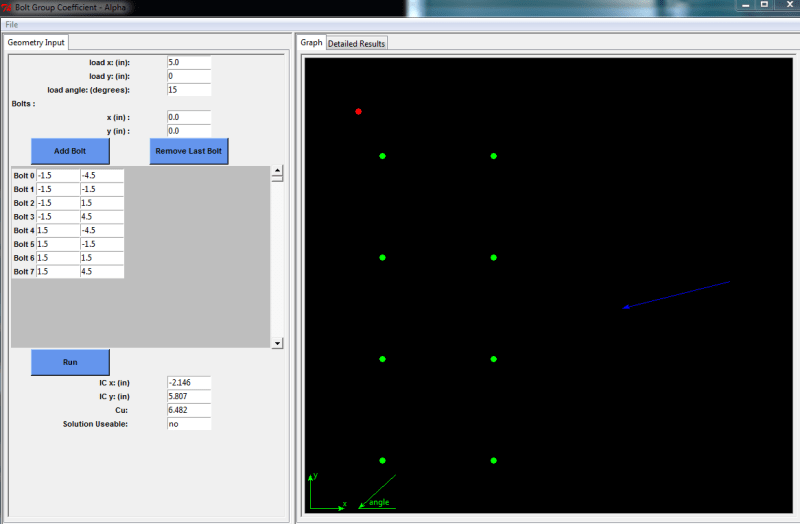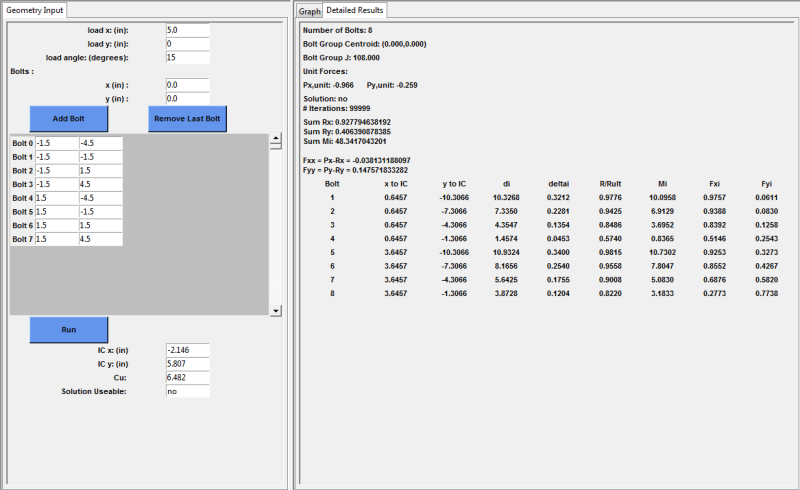Open Source Structural Applications: https://github.com/buddyd16/Structural-Engineering

### RE: Python Solve Functions - Help For A Noobie

It was a tolerance issue certain bolt and load configurations don't converge beyond a certain precision. I added the ability to control the tolerance.

the 8 bolt group needed a tolerance of 0.00001
a 3 bolt group (0,0) (0,3) (0,6) load at (5,3) angle: 15 needed a tolerance of 0.1 on my end

I'm sure the scipy module may have something built in to handle these odd conditions.

Open Source Structural Applications: https://github.com/buddyd16/Structural-Engineering

### RE: Python Solve Functions - Help For A Noobie

Double precision is the default for floating point numbers in Python (and Excel).

Doug Jenkins
Interactive Design Services
http://newtonexcelbach.wordpress.com/

### RE: Python Solve Functions - Help For A Noobie

Celt83 - I'm getting good convergence with your example, using eccentricity = 5 and Theta = 75. In my code Theta is the angle to the vertical, but it works with Theta = 15 as well. I can set the tolerance down to 1E-13, and it still converges in 1.5 milliseconds. My results (with Theta = 75) are:
ICx = -0.662, ICy = -3.0299, Pu = 95.2908 (see screen-shot below).

One possible reason for the difference in results is that my code takes the eccentricity as being the perpendicular distance from the centroid of the bolt group to the line of action of the applied force, as shown in the diagrams in the first link posted by sticksandtriangles. You seem to be using an XY coordinate for the point of application, with Y = 0, and that would give a different eccentricity for any non-vertical load.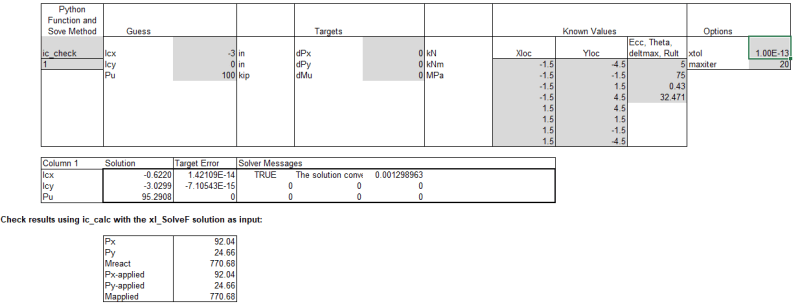Doug Jenkins
Interactive Design Services
http://newtonexcelbach.wordpress.com/

### RE: Python Solve Functions - Help For A Noobie

@IDS: think you may be right on the eccentricity side I'm trying to align with AISC table 7-8 which puts the load at an ex eccentricity with the load y coordinate aligned with the bolt group centroid.

Edit:think the equivalent e on your end would be 1.2941

I added a convergence graph to my gui here is what happens to my version of the Brandt algorithm if I keep the precision too tight, 0.000001: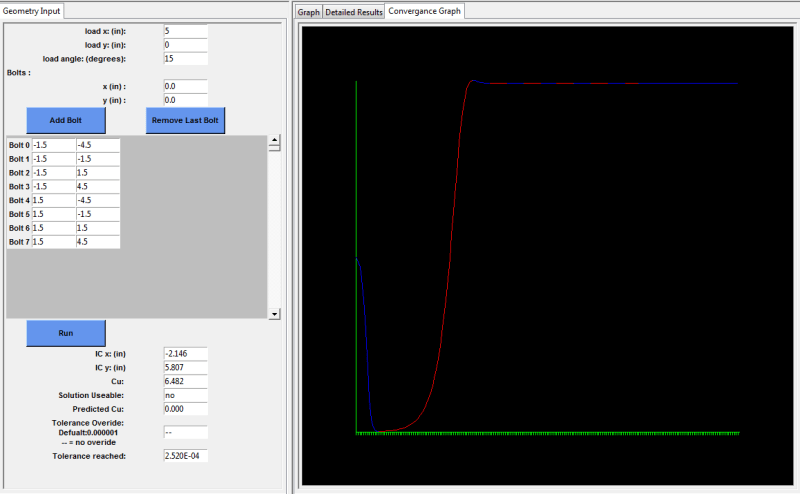vs a tol=0.001: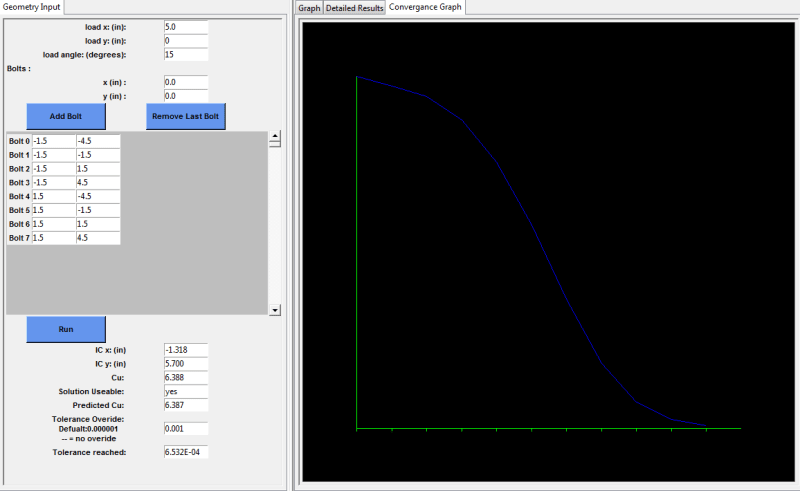Open Source Structural Applications: https://github.com/buddyd16/Structural-Engineering

### RE: Python Solve Functions - Help For A Noobie

(OP)
So I had this working and now my python program is getting a little finicky.

Now when I run the program i get an error that states "import scipy.optimize as sci" no module named optimize.

Any thoughts on what happened (it was working before)? I do not even know where to check to see where this going wrong. It looks like my console is no longer the same, so I might have moved directories or something?

S&T

(OP)

S&T

### RE: Python Solve Functions - Help For A Noobie

Seems you got it sorted was going to suggest re-installing.

I've added another chart showing the stability of the C factor across the calculation iterations. This can aid in making an educated decision on whether the smaller tolerances needed sometimes result in a C that is relatively stable or not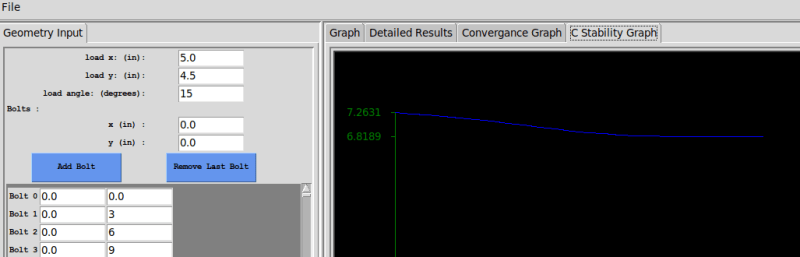Open Source Structural Applications: https://github.com/buddyd16/Structural-Engineering

### RE: Python Solve Functions - Help For A Noobie

Yakpol has a good spreadsheet for this calculation, with detailed output and also a theory page:
http://yakpol.net/BoltGroup_down.html

It uses the Excel Solver, but works pretty fast. It is set up with exactly same example as we are working on, except the factored bolt shear strength (Phi.Rn) is set to 27.06 kip.

To check against my code I set Ru to 27.06/0.75, and I corrected deltamax to 0.34 in (I had entered it as 0.43 in in the examples posted previously). With Y set to zero in the Yakpol spreadsheet the eccentricity for my code = X*cos(theta), where theta is the angle to the vertical. With those changes my results agree exactly with Yakpol's spreadsheet.

Celt83 - I tried your code, and using your ic_brandt function with final Icx and Icy values I get exact agreement with the first 4 results in the table output, but all force and moment results are lower by the Ru factor. I don't understand why you are treating Ru as a variable.

Compared with your brandt function, my results are completely different. Have you made significant changes to the posted code?

Finally a warning - first hit on my searches was a site called engineersviewpoint.blogspot. This seems to have been hijacked. I clicked on a link to an updated version of a post supposedly on Brandt's Method with VBA and immediately got pop-up messages that my hard drive was about to be erased.

Doug Jenkins
Interactive Design Services
http://newtonexcelbach.wordpress.com/

### RE: Python Solve Functions - Help For A Noobie

IDS:

Edit: oh all the fxi and fyi's will be off by Ru I did that multiplication at the end so not in the table values.I ment to get that corrected so it shows in the per bolt force table.

Edit2: this may be relevent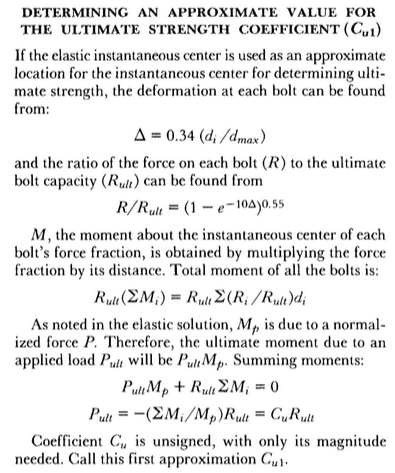with the approach of Pult=1, then Rult = -Mp/sumMi, so my Mi table won't match yours but I've fixed the Fxi and Fyi table values to include Rult now.

Open Source Structural Applications: https://github.com/buddyd16/Structural-Engineering

### RE: Python Solve Functions - Help For A Noobie

IDS:

I misread your post, Rult is a variable as it is based on the bolt diameter and material. My current functions calculate everything relative to the ratio of the bolt forces to the ultimate force. Doing it this way you get the C multiplier for that bolt arrangement and can then back into a bolt size/material.

Open Source Structural Applications: https://github.com/buddyd16/Structural-Engineering

### RE: Python Solve Functions - Help For A Noobie

I solved my issue, with very small eccentricities the ax, ay step size proposed by Brandt ends up being too large and you get to an equilibrium of over/under shooting the actual IC. Using the same equations for ax, and ay but dividing them in half or by 10 takes more iterations to find the IC but lowers the risk of overshoot.

Open Source Structural Applications: https://github.com/buddyd16/Structural-Engineering

### RE: Python Solve Functions - Help For A Noobie

(OP)
so, ive done a complete removal of python xy on my work computer and reinstall and I still get an error:

#### CODE --> Python

import scipy.optimize as sci
yields:

#### CODE --> python

ImportError: No module named optimize

Not sure what the hang up is. I have this working on my home computer and I just installed python xy there and it works like a charm. Any help would be appreciated.

Thanks,
S&T

S&T

### RE: Python Solve Functions - Help For A Noobie

you could try opening a command prompt ( windows key --> type cmd.exe --> hit enter) then type pip install scipy.

pip is a package manager used with python and should have been installed if you did a default install of python xy.

Open Source Structural Applications: https://github.com/buddyd16/Structural-Engineering

### RE: Python Solve Functions - Help For A Noobie

S&T - You might like to look at installing Anaconda Python, which installs SciPy and Numpy by default, as well as other science and maths related packages. It also includes xlwings, which allows you to call Python functions from Excel, or vice versa.

Doug Jenkins
Interactive Design Services
http://newtonexcelbach.wordpress.com/

### RE: Python Solve Functions - Help For A Noobie

second IDS's suggestion Python XY is a bit older and the one I use out of convenience. Anaconda is the new "hot" item for installing a python bundle.

Open Source Structural Applications: https://github.com/buddyd16/Structural-Engineering

### RE: Python Solve Functions - Help For A Noobie

(OP)
thanks for the suggestions. I will try both of these options tomorrow

S&T

### RE: Python Solve Functions - Help For A Noobie

I added another tab to compute the AISC table values for verification of the method. I've run a bunch of them and have been getting results that match the results shown in AISC.

Open Source Structural Applications: https://github.com/buddyd16/Structural-Engineering

#### Red Flag This Post

Please let us know here why this post is inappropriate. Reasons such as off-topic, duplicates, flames, illegal, vulgar, or students posting their homework.

#### Red Flag Submitted

Thank you for helping keep Eng-Tips Forums free from inappropriate posts.
The Eng-Tips staff will check this out and take appropriate action.

#### Resources

White Paper - A Guide to 3D Printing Materials
When it comes to using an FDM 3D printer effectively and efficiently, choosing the right material at the right time is essential. This 3D Printing Materials Guide will help give you and your team a basic understanding of some FDM 3D printing polymers and composites, their strengths and weaknesses, and when to use them. Download Now
White Paper - Multidisciplinary Simulation and Design Exploration in the Chemical and Process Indust
Simcenter STAR-CCM+ makes simulation accessible to process engineers with limited simulation experience but strong process knowledge. This flood of knowledge and skills will make the industry more cost efficient, less polluting and ultimately more innovative. Download Now
White Paper - Multidisciplinary Simulation and Design Exploration in the Oil and Gas Industry
The need to reduce exploration and development costs has never been more important to the future of the oil and gas industry. In this special report we present how engineers and scientists from leading oil and gas companies are deploying Siemensâ€™ simulation software in the design and operation of a range of oil and gas products and systems. Download Now
Research Report - Driving Engineering-to-order Differentiation and Profitability
Customized products are more compelling to customers and drive higher profitability. This engineer-to-order (ETO) study finds that some companies have found better ways to design custom products, alleviating the engineering bottleneck created in most companies when quotes requests or orders roll in. Download Now

Close Box

# Join Eng-Tips® Today!

Join your peers on the Internet's largest technical engineering professional community.
It's easy to join and it's free.

Here's Why Members Love Eng-Tips Forums:

•Talk To Other Members
• Notification Of Responses To Questions
• Favorite Forums One Click Access
• Keyword Search Of All Posts, And More...

Register now while it's still free!